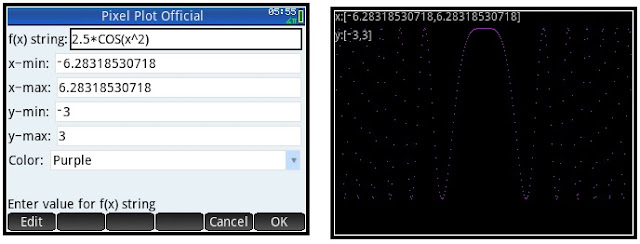## Tuesday, February 6, 2018

### HP Prime: Pixel Plot, How to Change Cartesian Coordinates to Pixels

HP Prime: Pixel Plot, How to Change Cartesian Coordinates to Pixels

Changing Cartesian Coordinates to Pixels

When running programs on the HP Prime, the screen has a pixel coordinate system of 320 x 220 (to allow room for soft menu keys).

There are two ways to calculate to translate Cartesian coordinates to pixel coordinates on the HP Prime.  The easy way is to use the CPX command.

However, if you are working in custom made apps, CPX may not work because the command requires that app has the Plot variables Xmin, Xmax, Ymin, and Ymax.  This will require a conversion formula.

Given a desired xmin, xmax, ymin, and ymax, the following formulas I use are:

Scaling:
xs = (xmax – xmin)/320
ys = (ymax – ymin)/-220 = (ymin – ymax)/220

Conversion to pixels of coordinates (x,y):
xp = (x – xmin)/xs
yp = (y – ymax)/ys

HP Prime Program: PIXELPLOT

EXPORT PIXELPLOT()
BEGIN
// EWS 2014-02-04

LOCAL xm,ym,xp,yp,x,y;
LOCAL xn,yn,xs,ys;
LOCAL ya,ch,flag;
LOCAL fx;

// set color scheme
LOCAL col1,col2;
col1:={#BF00FFh,#7DF9FFh,
#00FF00h,#D4AF37h,#FF0000h};
col2:={#4B0082h,#000080h,
#228B22h,#C3B091h,#800000h};

HAngle:=0;

INPUT({{fx,},
xm,xn,ym,yn,
{ch,
{"Purple","Blue","Green",
"Gold","Red"}}},
"Pixel Plot Official",
{"f(x) string:",
"x-min: ","x-max: ",
"y-min: ","y-max: ",
"Color: "});

RECT_P(0);

// calulate the scale
xs:=(xn-xm)/320;
ys:=(yn-ym)/−220;

// drawing

// color choice
LOCAL c1,c2;
c1:=col1[ch];
c2:=col2[ch];

// axis information
LOCAL st1,st2;
st1:="x:["+xm+","+xn+"]";
st2:="y:["+ym+","+yn+"]";
TEXTOUT_P(st1,0,0,2,#C0C0C0h);
TEXTOUT_P(st2,0,20,2,#C0C0C0h);

// function
FOR x FROM xm TO xn STEP xs DO

// skip 0 for now
IF x==0 THEN
CONTINUE;
END;

// function
y:=EXPR(fx);

// point→pixel, plot
// only if y is real
IF TYPE(y)==0 THEN
xp:=(x-xm)/xs;
yp:=(y-yn)/ys;
PIXON_P(xp,yp,c1);
END;

END;

// freeze screen
FREEZE;

END;

Notes:

1. You should not have to include the string characters for f(x) as they are included in the input.

2. Use the lowercase x.

3. The program errors if a plot reaches point where f(x) is not defined.  I put in a condition when f(x) is complex (for example, the square root of negative number) for the plot to skip that pixel.  However, I have not put error skipping conditions when it comes to ln(x) or 1/p(x) where p(x) is a polynomial.  However, the pixel at x=0 is skipped to hopefully alieve some problems.  Be sure your range is appropriate.

4. The program uses Radians angle mode (HAngle = 0).

5.  I chose to have a black background with five color options (purple, electric blue, green, gold, and red) just for fun.

Examples

Example 1:  f(x) = 2.5*cos(x^2)Example 2:  f(x) = √(x^2 – 6)Example 3:  f(x) = e^(-x^2)Eddie

This blog is property of Edward Shore, 2018.

### Casio fx-CG50: Sparse Matrix Builder

Casio fx-CG50: Sparse Matrix Builder Introduction The programs can create a sparse matrix, a matrix where most of the entries have zero valu...